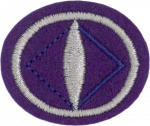## OpticsSkill Level:  2
Original Honor:  1962
Originating Institution: General Conference

Requirements:

1. Define and/or draw a diagram of the following:

a. Focal length

b. Positive lens

c. Negative lens

d. Two kinds of distortion

e. Longitudinal color

f. Lateral color

g. Spherical aberration

h. Achromatic lens

i. Refraction of light

2. Explain how light behaves when it strikes or traverses water, oil, feldspar, and a mirror.

3. Name and draw diagrams of three kinds of positive lenses and three kinds of negative lenses.

4. What should be the minimum distance of light source from the lens when testing for focal length?

5. Find the focal length of at least four lenses, one being a negative lens.

6. Explain by diagram why an image from a positive lens makes an image reversed and inverted.

7. Show with diagrams how a prism works. State the angles at which the colors appear and disappear.

8. Show and demonstrate what happens when light strikes one-way glass.

9. Construct one optical instrument using mirrors or lenses, such as a periscope, a slide or opaque projector, or a simple telescope.

10. Explain what is meant by the term 6x35 and 7x50 as applied to binoculars.

11. Define the term "f/stop" as used in connection with cameras. What does it mean when a lens is fast or slow? Is an f/8.5 lens faster or slower than an f/8 lens?